# Physics 1.5: Thermodynamics Flashcards Preview

## MCAT > Physics 1.5: Thermodynamics > Flashcards

Flashcards in Physics 1.5: Thermodynamics Deck (30):
1

2

3

4

5

6

7

8

9

10

11

12

13

14

15

16

17

18

19

20

21

## Work is pathway dependent

### Calculate the work done on or by a system by finding the area under the pressure-volume curve. V constant, with  ΔP : then no work is done (because there is no area to calculate.)  P constant , with  ΔV:  the area under the curve is a rectangle of length P and width Δ V (Vf− Vi). Work is as follows:22

## What work is done when decrease in pressure from P1 to P2 at a constant volume?

### no change in volume, the work done in this process is zero.(Isochoric)23

## What work is done when system expands from V1 to V2 at a constant pressure?

### isobaric process(+)W = PΔ V.Because the gas has expanded, the work was done by the gas and is positive.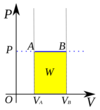24

## What work is done when neither pressure nor volume is held constant?

### graphical analysis (fig C): The total area under the graph (Regions I and II) gives the work done The work done is the sum of the areas of regions I and II: W=A1 + A2 Region I is a triangle whose base is Δ V and whose height is Δ P, so the area is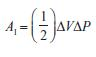Region II is a rectangle with base Δ V and height P2, so its area is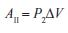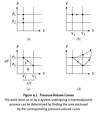25

## What work is done in a closed cycle in which, after certain interchanges of work and heat, the system returns to its initial state?

### Because work is positive when the gas expands and negative when the gas is compressed, the work done is the area enclosed by the curve.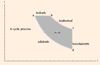26

## three particular thermodynamic processes as special cases of the first law

### isovolumetric, isochoric; (constant volume),Q=0; ΔU=Q adiabatic (no heat exchange): W=0; ΔU= -W closed cycle, isothermal,  (constant internal energy, return to initial state), ΔU=0, Q=W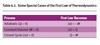27

28

29

30